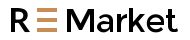Free Shipping on orders over US\$39.99

# The most commonly used PLC input and output methods: switch quantity and analog quantity

Switch quantity and analog quantity are the two most commonly used input and output methods in the early stage of learning PLC. What is switch quantity? What is an analog quantity? This question must be clarified.

Switch quantity and analog quantity are the two most commonly used input and output methods in the early stage of learning PLC. What is switch quantity? What is an analog quantity? This question must be clarified.Figure 1 is a typical device that can output switching signals.

When the pressure is high, the two contacts of C and B are closed and connected, and the high pressure signal is output. When the pressure is low, the two contacts of C and A are closed and connected, and the low pressure signal is output. With such a signal, the local pressure signal can be transmitted to the remote electrical control cabinet to participate in automatic remote control, where C and B are a switch, and C and A are also a switch. So a switch contact is a switch, and its characteristic is that it is either on or off at the same time. On is 1, which means there is a signal, and off is 0, which means no signal. This is the so-called switching signal.

Although the pressure gauge can transmit the pressure signal to a distance, it only transmits the signal of whether there is pressure, and it is impossible to know what the real-time pressure value is.The device in Figure 2 is called a pressure transmitter.

The inside of the pressure transmitter is a circuit board, which is connected to a pressure sensor F. Its working principle is that the pressure sensor F transmits the detected pressure to C of the circuit board. After the detection signal enters the circuit board, through the conversion and calculation of the circuit board, the pressure signal is converted into a current signal by A and B. point output. The right side of the figure is the schematic diagram of the conversion process. It can convert a 0-10kpa pressure signal into a 4-20mA current signal, which is output by the two points A and B. At this time, we say that the output of the two points A and B is an analog signal. The characteristic of an analog signal is that its value is continuously variable within a range of values.

Let’s take a look at how analog signals are transmitted over long distances.A pressure transmitter with a range of 0-10kpa is installed on our pipeline. The positive pole of the power supply is connected to point B of the pressure transmitter, and the negative pole is connected in series with a multimeter to point A of the pressure transmitter, and the multimeter is switched to the current gear. When the pressure at point C of the pressure transmitter is 5kpa, the current reading of the multimeter is 12mA. It is exactly the middle value of the current signal of 4-20mA, and 5kpa is also the middle value of the pressure value of 0-10kpa. When the pressure at point C of the pressure transmitter is 10kpa, the current reading of the multimeter is exactly 20mA. In this way, the pressure value of 0-10kpa corresponds to the current signal value of 4-20mA. We only need to extract the current signal value of 4-20mA through a receiving device in the distance, and then through certain calculations, we can know the local pressure. What is the value.

Why convert the pressure signal into a 4-20mA current signal instead of a 0-20mA current signal or a 0-10V voltage signal?

The voltage signal of 1.0-10V is susceptible to electromagnetic interference from the outside world, especially when the cable length is very long.

2. If the current signal of 0-20mA is used, it is impossible to judge when the current signal is 0mA, whether it is the fault 0mA caused by the cable disconnection, or the normal 0mA output by the pressure itself being 0kpa.Figure 4 is a wiring diagram of using Siemens S7-200 PLC to read the pressure value of the pressure transmitter. This is the most basic method of use. The left side is the switch quantity, and the right side is the analog quantity. Different signal types need to be connected to PLC different inputs.